# waynehu

## Professor, Department of Astronomy and Astrophysics University of Chicago

Group Contact CV SnapShots

## Cosmological Parameter Estimation

The huge advantage of bandpowers is that they represent the natural meeting ground of theory and experiment. The above two sections outline some of the steps involved in extracting them from the observations. Once they are extracted, any theory can be compared with the observations without knowledge of experimental details. The simplest way to estimate the cosmological parameters in a set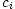is to approximate the likelihood as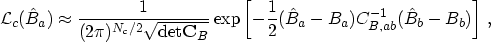(35)

and evaluate it at many points in parameter space (the bandpowers depend on the cosmological parameters). Since the number of cosmological parameters in the working model is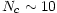this represents a final radical compression of information in the original timestream which recall has up to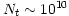data points.

In the approximation that the band power covariance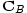is independent of the parameters, maximizing the likelihood is the same as minimizing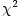. This has been done by dozens of groups over the last few years especially since the release of CMBFAST [Seljak & Zaldarriaga, 1996], which allows fast computation of theoretical spectra. Even after all the compression summarized in Figure 5, these analyses are still computationally cumbersome due to the large numbers of parameters varied. Various methods of speeding up spectra computation have been proposed [Tegmark & Zaldarriaga, 2000], based on the understanding of the physics of peaks outlined in §3, and Monte Carlo explorations of the likelihood function [Christensen et al, 2001].

Again the inverse Fisher matrix gives a quick and dirty estimate of the errors. Here the analogue of Equation (29) for the cosmological parameters becomes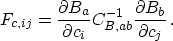(36)

In fact, this estimate has been widely used to forecast the optimal errors on cosmological parameters given a proposed experiment and a band covariance matrix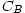which includes diagonal sample and instrumental noise variance. The reader should be aware that no experiment to date has even come close to achieving the precision implied by such a forecast!

As we enter the age of precision cosmology, a number of caveats will become increasingly important. No theoretical spectra are truly flat in a given band, so the question of how to weight a theoretical spectrum to obtain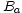can be important. In principle, one must convolve the theoretical spectra with window functions [Knox, 1999] distinct from those in Equation (30) to produce. Among recent experiments, DASI [Pryke et al, 2001] among others have provided these functions. Another complication arises since the true likelihood function foris not Gaussian, i.e. not of the form in Equation (35). The true distribution is skewed: the cosmic variance of Equation (4) leads to larger errors for an upward fluctuation than for a downward fluctuation. The true distribution is closer to log-normal [Bond et al, 2000], and several groups have already accounted for this in their parameter extractions.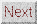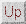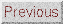Next: DISCUSSION Up: DATA ANALYSIS Previous: Bandpower Estimation
Wayne Hu 2001-10-15